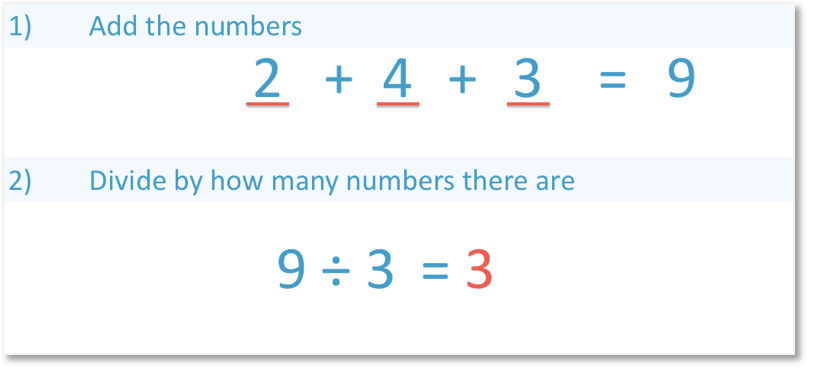# How to Calculate a Ratio of 3 Numbers

How to Calculate a Ratio of 3 Numbers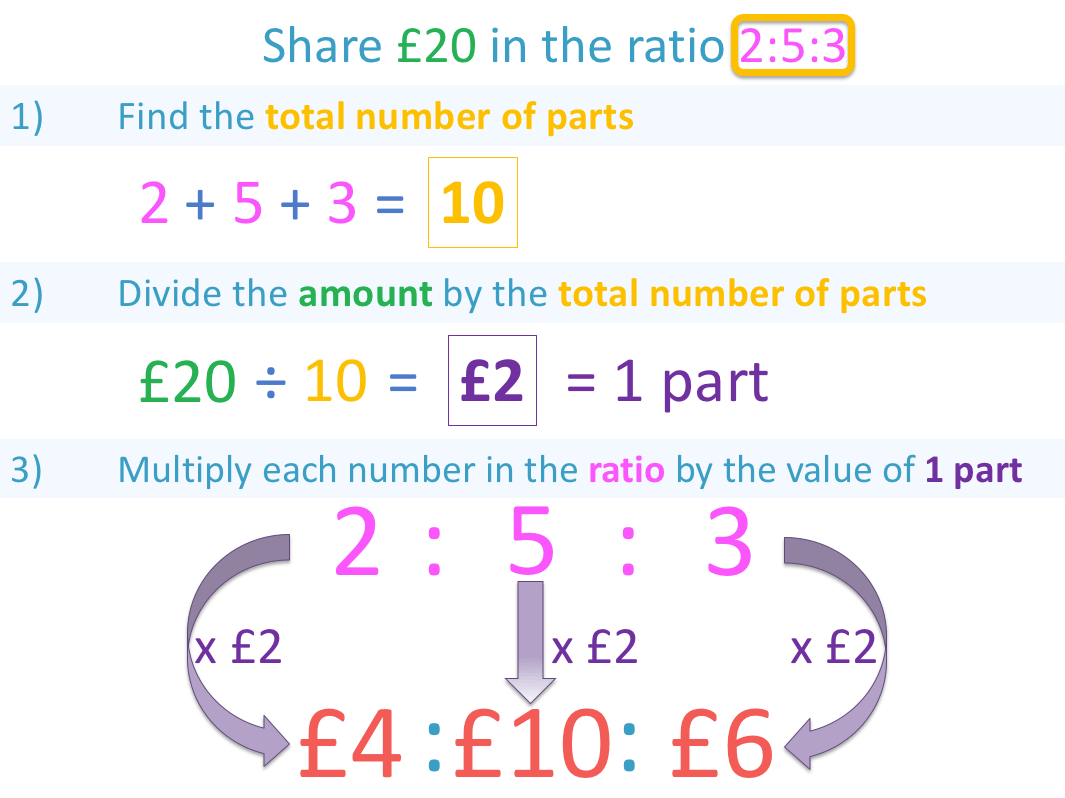• There are three numbers in the ratio: ‘2’, ‘5’ and ‘3’. This means that the amount is shared between three people.
• 2 + 5 + 3 equals a total of 10 parts in the ratio.
• To find the value of each part, we divide the amount of £20 by the total number of parts.
• £20 ÷ 10 = £2.
• Finally we multiply each of the three numbers in our ratio by the value of each part.
• 2:5:3 multiplied by £2 gives the final answer of £4:£10:£6.
The value of each part is found by dividing the given amount by the sum of the parts in the ratio.

We then multiply each number in the ratio by the value of each part.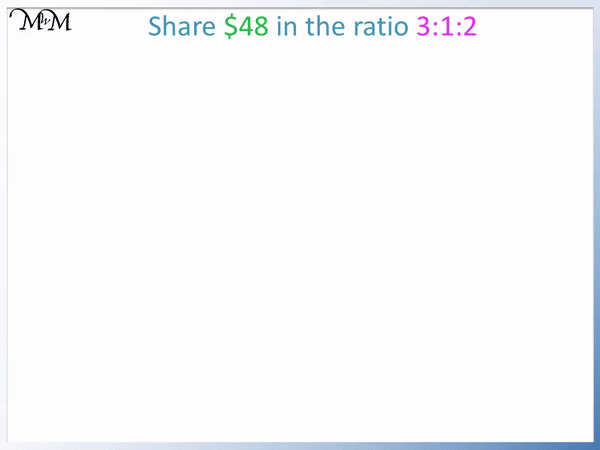• We are sharing $48 between three people in the ratio 3:1:2. • The total number of parts in the ratio is 3 + 1 + 2 = 6 parts. • To find the value of each part in the ratio we divide$48 by the total number of parts.
• $48 ÷ 6 =$8.
• To find how much each person receives we multiply the ratio by the value of each part.
• 13:1:2 multiplied by $8 is$24:$8:$16.
• The first person receives $24, the second person receives$8 and the third person receives $16.Supporting Lessons#### Calculating a Ratio of 3 Numbers: Interactive Questions #### Sharing in a Ratio of 3 Numbers: Worksheets and Answers # How to Calculate Ratios of 3 Numbers The amount of numbers in a ratio tell us how many people we are sharing an amount between. In this lesson we will be looking at calculating ratios that contain 3 numbers and so, we will be sharing a given amount between three people. To calculate a ratio of 3 numbers, we follow 3 steps: • Step 1: Find the total number of parts in the ratio by adding the numbers in the ratio together • Step 2: Find the value of each part in the ratio by dividing the given amount by the total number of parts. • Step 3: Multiply the original ratio by the value of each part Here is our first example of calculating a ratio of three numbers:We are asked to share$48 between three people in the ratio 3:1:2.

This means that for every three parts a person receives, a second person is given one part and a third person is given two parts.

We will work out how much money each person receives by following the three steps.

• Step 1: Find the total number of parts.Looking at the ratio 3:1:2, we have:

3 + 1 + 2 = 6

So, six parts in total.

• Step 2: Divide the given amount by the total number of parts in the ratio.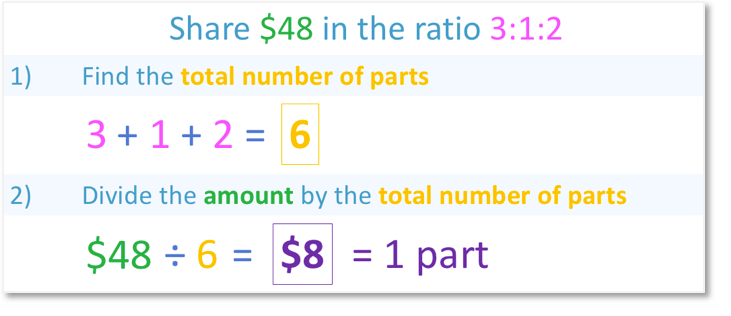The amount is $48 and the total number of parts is 6. 48 ÷ 6 = 8 Each part in the ratio is worth$8.

• Step 3: Multiply each number in the ratio by the value of one part.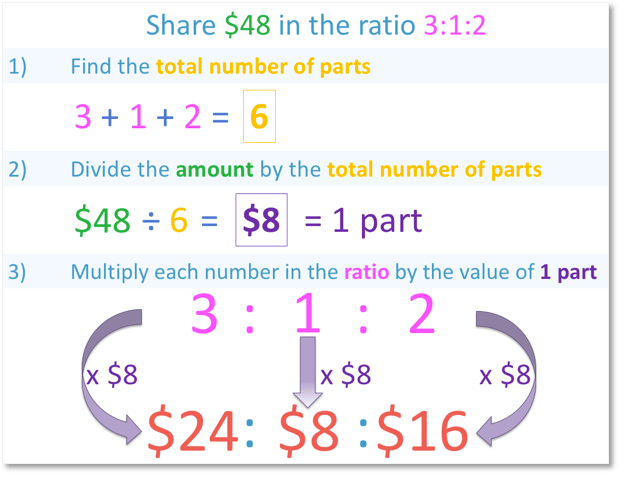We will multiply each number in the ratio 3:1:2 by $8. 3 x$8 = $24 1 x$8 = $8 2 x$8 = $16 The money is shared in the ratio$24:$8:$16.

The first person receives $24, the second person receives$8 and the third person receives $16. Having calculated this ratio of three numbers, we can check our result by adding the values together.$24 + $8 +$16 = \$48, which is the total amount that we started with.

We can also check our result by simplifying the ratio 24:8:16.

All of these numbers are in the eight times table and dividing them by 8, we get back to our original ratio of 3:1:2.Now try our lesson on Finding the Mean where we learn how to find the mean of a set of numbers.